# Ashutosh Writes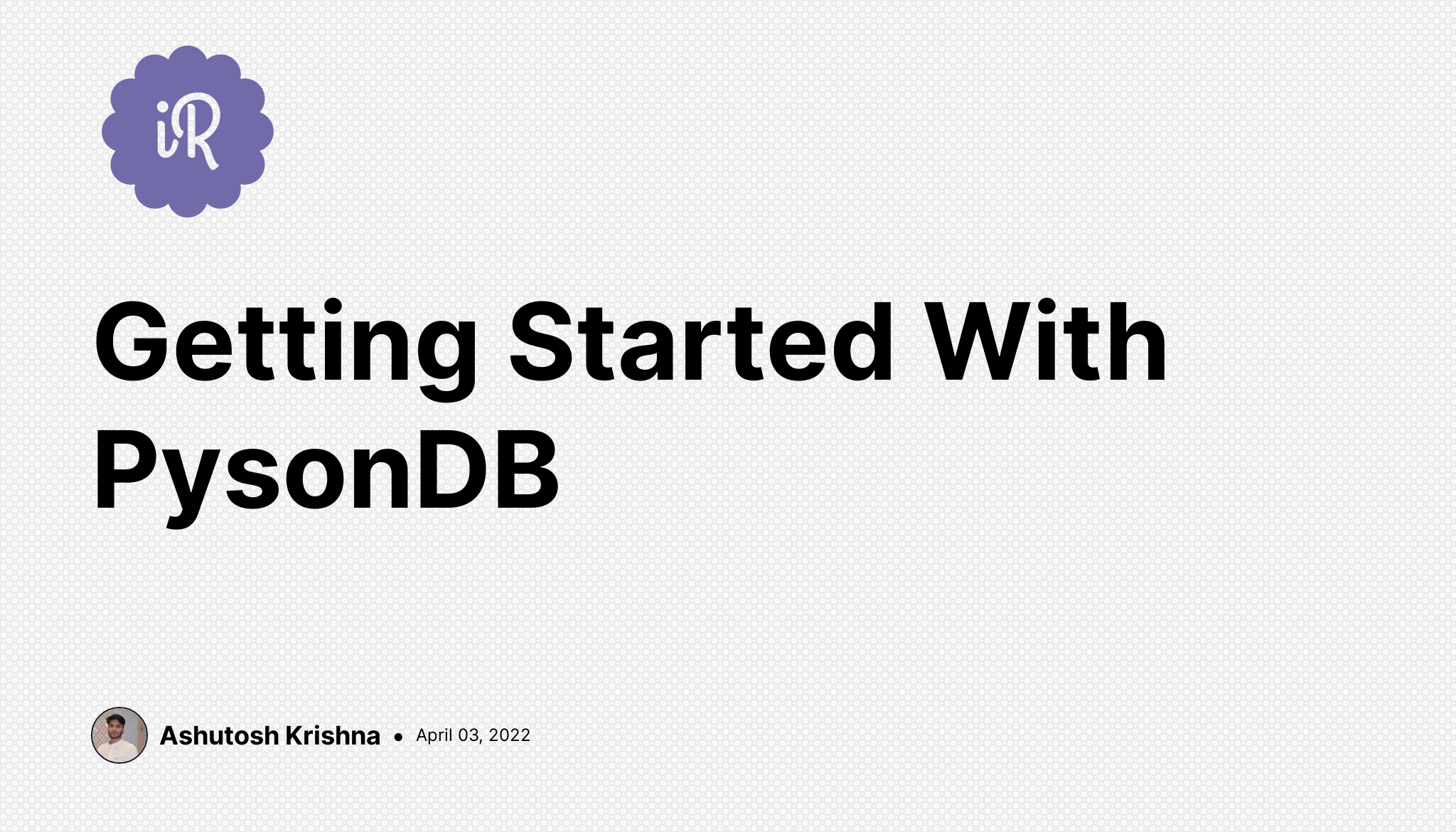# Getting Started With PysonDB

## Introduction

We often need to store some data while working on our personal or minor projects. We can use a SQL or NoSQL database with a server, but that would require us to do a bit of setup. In one of the previous blogs, we had seen a solution to this problem with the use of TinyDB.

But we are not going to discuss TinyDB in this blog. If you're interested in learning about it, check out this blog. In this blog, we're going to discuss another similar solution to this problem using PysonDB.

## What is PysonDB?

PysonDB is yet another document-oriented database written in pure Python. Developed by Fredy Somy, it is simple, lightweight, and efficient.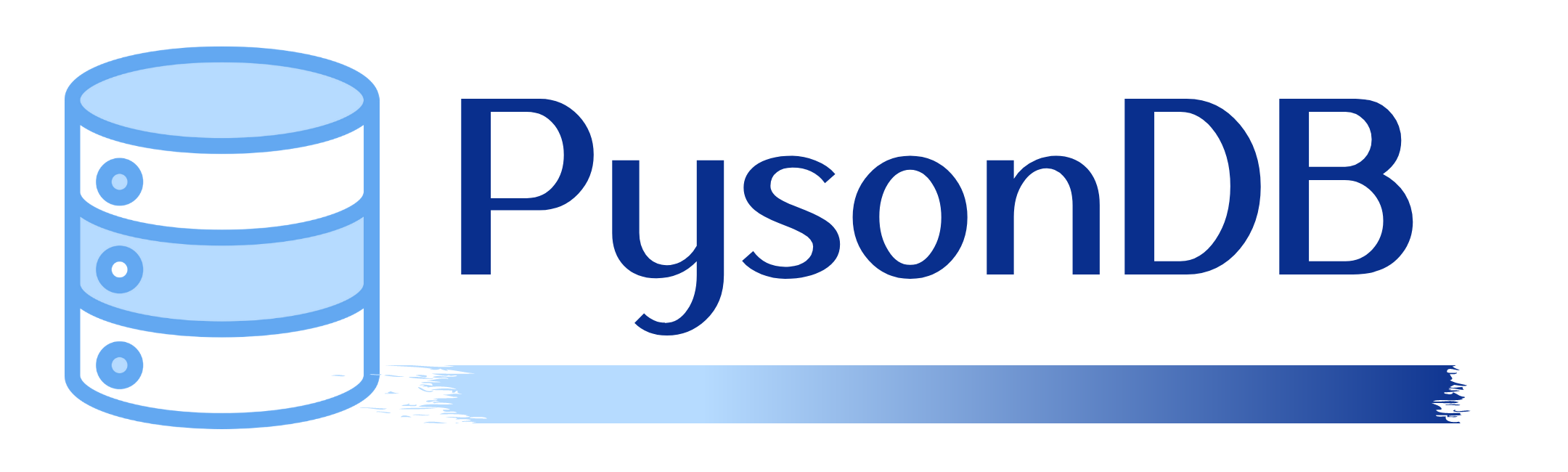The word PysonDB is built of two words: Python and JSON (and of course, DB! 😉. Thus it is a JSON-based database.

It has a lot of features like:

• It is lightweight and JSON-based.
• It supports CRUD operations.
• No database drivers are required.
• A unique ID is assigned to each JSON document added automatically.
• It has an in-built CLI to delete, display and, create the database.

Note: You cannot store images, videos, etc.

# How to Install PysonDB

It is extremely easy to install PysonDB. Just run this command in your terminal:

``````pip install pysondb
``````

## How to Use PysonDB

Similar to the TinyDB tutorial, let us consider an example of a Todo Application where we just need to perform CRUD operations. Now that we have PysonDB installed, let's see how we can use it.

The very first thing we will do is create a database called `todo.json`. It's quite easy to do it using PysonDB.

``````from pysondb import getDb

todo_db = getDb('todo.json')
``````

We just need to call the `getDb()` method with the JSON filename and when you run the file, it will automatically create an empty database (JSON file) for you.

``````{ "data": [] }
``````

## How to Insert Data

Inserting data is quite simple in PysonDB. We have two methods - `add()` to insert one object and `addMany()` to obviously add more than one. The only thing we need to take care of is the schema of the database. Whatever data you add first, becomes the schema for the whole database. Any schema irregularity rejects the irregular data. If you didn't understand, let us understand it with an example.

``````new_item = {"name": "Book", "quantity": 5}
print(item_id)

## Output
## 259596727698286139
``````

First of all, we created a new dictionary called `new_item` with `name` and `quantity` set to `Book` and `5`, respectively. Then we used the `add()` method to insert the data into our database. The `add()` method returns the unique ID of the object inserted.

Let us see how our JSON file looks like:

``````{
"data": [
{
"name": "Book",
"quantity": 5,
"id": 259596727698286139
}
]
}
``````

Now let us see an example of schema irregularity. Till now we have inserted an object with name and quantity fields. But now let's add another field price to it and try to add the data.

``````another_item = {"name": "Milk", "quantity": "5L", "price": 310}
print(another_item_id)
``````

Now if you try to run the program, you will encounter a `SchemaError`.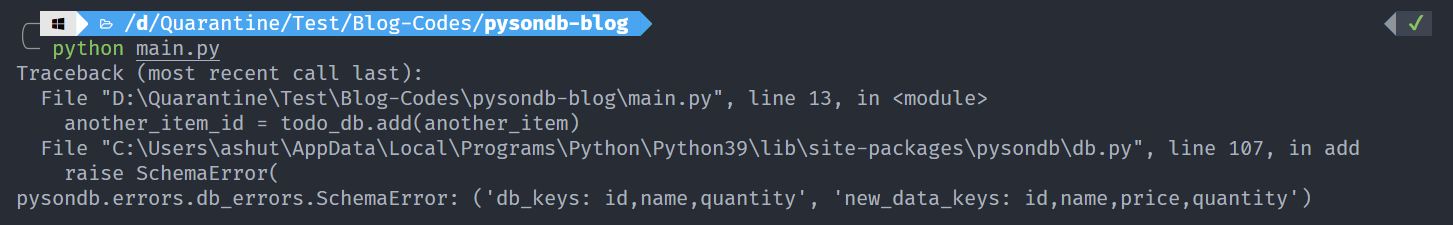Hope the statement is now clear.

Now, let us see how we can add more than one object using the `addMany()` method.

``````new_items = [
{"name": "Copies", "quantity": 10},
{"name": "Pen", "quantity": 4},
]
``````

In this case, we created a list of dictionaries called `new_items` and used the `addMany()`method to insert the items. This method doesn't return anything.

In this case too, we can face schema irregularity problem.

``````other_new_items = [
{"namme": "Dictionary", "quantity": 1},
{"name": "Stickers", "quantity": 10},
]
``````

We have misspelled the name field as namme, and thus we will encounter the `SchemaError`.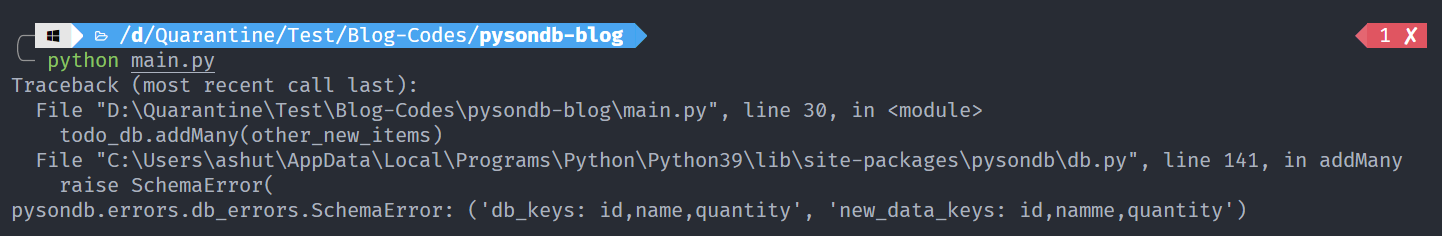## How to Retrieve Data

There are several methods to retrieve data from the database. Let's look at them one by one.

#### get()

The `get()` method by default returns one item from the database.

``````data = todo_db.get()
print(data)
``````

Output:

``````[{'name': 'Book', 'quantity': 5, 'id': 259596727698286139}]
``````

The `get()` method takes an optional parameter n where n is the number of objects to be retrieved.

``````data = todo_db.get(2)
print(data)
``````

Output:

``````[{'name': 'Book', 'quantity': 5, 'id': 259596727698286139}, {'name': 'Copies', 'quantity': 10, 'id': 313160125004626021}]
``````

However, if we give a value of n more than the number of objects in the database, it returns a list with a dictionary having an empty string as key as well as value.

``````data = todo_db.get(10)
print(data)
``````

Output:

``````[{'': ''}]
``````

#### getAll()

As the name itself suggests, it will return all the data from the database.

``````data = todo_db.getAll()
print(data)
``````

Output:

``````[{'name': 'Book', 'quantity': 5, 'id': 259596727698286139}, {'name': 'Copies', 'quantity': 10, 'id': 313160125004626021}, {'name': 'Pen', 'quantity': 4, 'id': 588928180640637551}]
``````

#### getByQuery()

The `getByQuery()` method takes a parameter query where query itself is JSON data. It returns data matching the query.

``````q = {"name": "Book"}
data = todo_db.getByQuery(query=q)
print(data)
``````

Output:

``````[{'name': 'Book', 'quantity': 5, 'id': 259596727698286139}]
``````

If no data matches the query, an empty list is returned.

Note: This method is a replacement for `getBy(query)` method which will be deprecated soon.

#### getById()

If you wish to get an object using its unique ID, `getById()` method will come handy. It takes an integer parameter pk which is the unique ID given to each object in the database.

``````data = todo_db.getById(pk=588928180640637551)
print(data)
``````

Output:

``````{'name': 'Pen', 'quantity': 4, 'id': 588928180640637551}
``````

If no id matches the provided id, we encounter a `IdNotFoundError`.

``````data = todo_db.getById(2)
print(data)
``````

Output: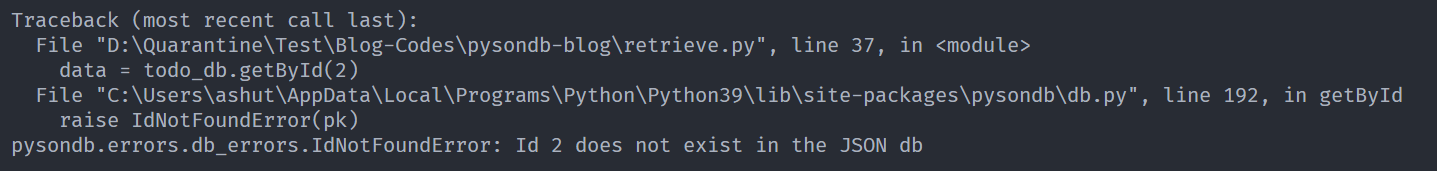Note: This method is a replacement for `find(id)` method which will be deprecated soon.

#### reSearch()

The `reSearch()` method takes two parameters: key and _re where key is any of the keys from the database such as name and quantity in our example, and _re is a regex pattern for the value of the respective key.

``````data = todo_db.reSearch(key="name", _re=r"[A-Za-z]*")
print(data)
``````

Here we have used a regex pattern for any word containing alphabets and the key is name.

``````[{'name': 'Book', 'quantity': 5, 'id': 259596727698286139}, {'name': 'Copies', 'quantity': 10, 'id': 313160125004626021}, {'name': 'Pen', 'quantity': 4, 'id': 588928180640637551}]
``````

## How to Update Data

To update data in PysonDB, we have three methods.

#### updateById()

The `updateById()` method takes two parameters: pk and new_data, where pk is the unique ID of the object which has to be updated with the new_data.

``````updated_data = {"name": "Book", "quantity": 100}
todo_db.updateById(pk=259596727698286139, new_data=updated_data)
``````

Output:

``````{
"data": [
{
"name": "Book",
"quantity": 100,
"id": 259596727698286139
},
{
"name": "Copies",
"quantity": 10,
"id": 313160125004626021
},
{
"name": "Pen",
"quantity": 4,
"id": 588928180640637551
}
]
}
``````

If the pk value doesn't exist, we get an `IdNotFoundError`.

#### updateByQuery()

The `updateByQuery()` method takes two parameters: db_dataset and new_dataset, where db_dataset refers to the query which needs to be changed with the new_dataset.

``````query_data = {"name": "Copies"}
updated_data = {"name": "Copies", "quantity": 200}
todo_db.updateByQuery(db_dataset=query_data, new_dataset=updated_data)
``````

Output:

``````{
"data": [
{
"name": "Book",
"quantity": 100,
"id": 259596727698286139
},
{
"name": "Copies",
"quantity": 200,
"id": 313160125004626021
},
{
"name": "Pen",
"quantity": 4,
"id": 588928180640637551
}
]
}
``````

If the query doesn't exist, we get a `DataNotFoundError`.

## How to Delete Data

We have two methods to delete data from the database.

#### deleteById()

It takes a parameter pk which by now you might already know it is. If not, pk is the unique ID of any data in the database.

``````is_deleted = todo_db.deleteById(pk=259596727698286139)
print(is_deleted)
``````

Output:

It returns whether the data was deleted or not.

``````True
``````

If not data matches the pk, we get `IdNotFoundError`.

#### deleteAll()

You might have already guessed what this method would do. It will clear the database.

``````todo_db.deleteAll()
``````

When executed, our data will be deleted from the database.

``````{ "data": [] }
``````

## Command Line Operations with PysonDB

One of the unique features of PysonDB is the command line operations that we can perform using it. Let us see what all we can do using the command line.

#### 1. Create Database

pysondb create `[name]`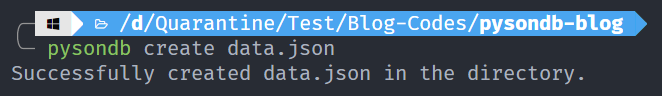This command helps us to create a database using the command line.

#### 2. Delete Database

pysondb delete `[name]`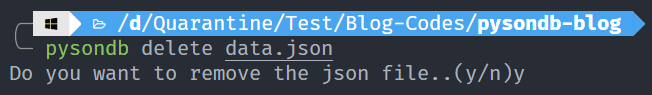This command helps us to delete an already existing database using the command line.

#### 3. Show Data

pysondb show `[name]`

We have a database called `todo.json` with the contents:

``````{
"data": [
{
"name": "Book",
"quantity": 5,
"id": 241737821309633823
},
{
"name": "Copies",
"quantity": 10,
"id": 895733868606022035
},
{
"name": "Pen",
"quantity": 4,
"id": 314476424041647076
},
{
"name": "Dictionary",
"quantity": 1,
"id": 338909711735495602
},
{
"name": "Stickers",
"quantity": 10,
"id": 460456836143359145
}
]
}
``````

Let us display this data.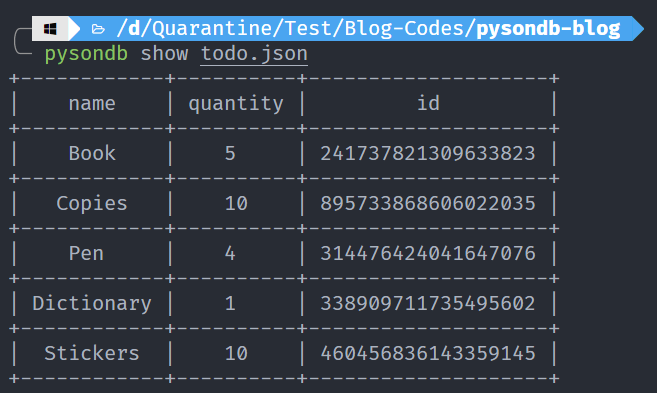Looks cool, doesn't it?

#### 4. Convert CSV Data to JSON

pysondb convert `[path of csv file]` `[path of json file]`

This command helps us convert CSV data into JSON database.

For example,we have a CSV file with the contents:

``````name,quantity,id
Book,5,241737821309633823
Copies,10,895733868606022035
Pen,4,314476424041647076
Dictionary,1,338909711735495602
Stickers,10,460456836143359145
``````

Let's convert it.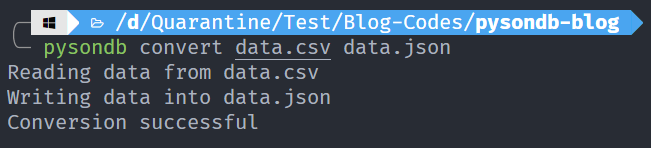#### 5. Convert JSON database to CSV data

pysondb converttocsv `[path of json file]` `[optional name for target CSV file]`

This command helps us convert JSON database to CSV data.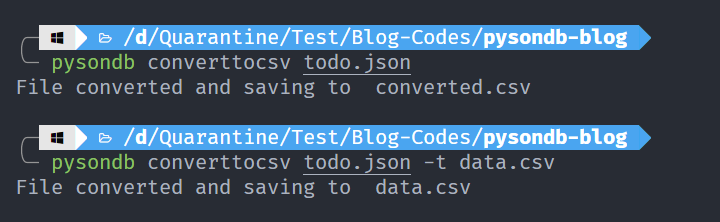Note: To specify custom path for CSV file, use the -t flag and then the CSV file path. See the second example above.

#### 6. Merge two JSON files

pysondb merge `[path of primary json file] [path of json file to merge]` `[optional name for target json file]`

We have two JSON files - one.json and two.json as.

one.json:

``````{
"data": [
{ "name": "Item1", "quantity": "5", "id": 9618007132 },
{ "name": "Item2", "quantity": "10", "id": 8052463398 },
{ "name": "Item3", "quantity": "4", "id": 1677865420 },
{ "name": "Item4", "quantity": "1", "id": 4466016920 },
{ "name": "Item5", "quantity": "10", "id": 9836191198 }
]
}
``````

two.json:

``````{
"data": [
{ "name": "Item6", "quantity": "5", "id": 9618007232 },
{ "name": "Item7", "quantity": "10", "id": 8052464398 },
{ "name": "Item8", "quantity": "4", "id": 1677865520 },
{ "name": "Item9", "quantity": "1", "id": 4466016020 },
{ "name": "Item10", "quantity": "10", "id": 9836181198 }
]
}
``````

The below command will merge data from two.json into one.json.

``````>>> pysondb merge one.json two.json
``````

Now our one.json file has the following contents:

``````{
"data": [
{ "name": "Item6", "quantity": "5", "id": 9618007232 },
{ "name": "Item7", "quantity": "10", "id": 8052464398 },
{ "name": "Item8", "quantity": "4", "id": 1677865520 },
{ "name": "Item9", "quantity": "1", "id": 4466016020 },
{ "name": "Item10", "quantity": "10", "id": 9836181198 },
{ "name": "Item1", "quantity": "5", "id": 9618007132 },
{ "name": "Item2", "quantity": "10", "id": 8052463398 },
{ "name": "Item3", "quantity": "4", "id": 1677865420 },
{ "name": "Item4", "quantity": "1", "id": 4466016920 },
{ "name": "Item5", "quantity": "10", "id": 9836191198 }
]
}
``````

Did you see the data from two.json is added on top of data in the one.json file?

We can also put the merged content into a separate file without changing the data of any of the files:

``````>>> pysondb merge one.json two.json -o merged.json
``````

The above command will create a merged.json file and put the merged content into that file. In this case, one.json and two.json will not be changed at all.

Notice the -o flag in the above command before the name of the output file.

## Conclusion

In this article, we have talked about PysonDB and how to perform CRUD operations on the database. We also saw how we can interact with PysonDB using the command line.